9 Appendix A - Horsepower Calculations — TaigDrives 2 documentation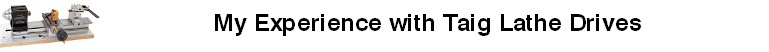#### Previous topic

8 Satisfaction at Last

#### Next topic

10 Appendix B - DC Motor Characteristics

# 9 Appendix A - Horsepower Calculations¶

Finding out how much power you need is not difficult. There are tables of unit power that define, for any material type, the horsepower needed to turn away one cubic inch per minute. If you’re turning metal and know the work diameter, depth of cut and feed rate, you can easily find how many cubic inches you’re removing per minute. Multiplying that value by the appropriate unit power factor gives the required horsepower. But I didn’t find all that information especially easy to read so here is my attempt to see the whole thing more clearly.

There are two major operations in lathe work, turning and facing. In both cases, the volume of material removed is a simple function of the following easy-to-measure variables:

• D = diameter of work in inches
• C = cutting depth in inches
• L = length of tool movement in inches
• T = time to move length L in minutes
• F = feed rate of tool in inches per minute =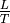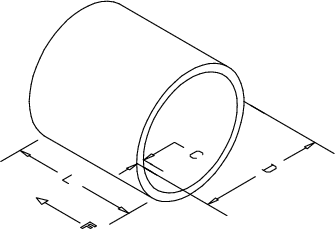Fig. 9.1.1: Turning Stock Removal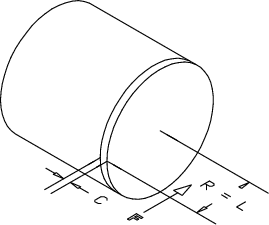Fig. 9.1.2: Facing Stock Removal

Turning reduces the diameter by removing a hollow cylinder as in Fig. 9.1.1. The volume removed is:(The actual formula for the volume of a hollow cylinder is slightly more complex but with small cutting depths the error is negligible and the simplified formula easier to apply in practice.) The rate of removal is: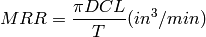Since the feed rate is, the material removal rate for turning is: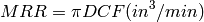Facing reduces the length by removing a solid cylinder as in Fig. 9.1.2. The volume removed is: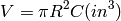The rate of removal is: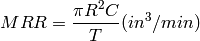Since the feed rate is=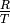the material removal rate for facing is:Of course, the facing rate is an average over a reducing radius and not really a magical halving of the turning rate. The instantaneous rate at any given radius is the same for both turning and facing. Cutting off is a special case of facing where the depth of cut is equal to the width of the parting tool.

Once you have the removal rate, a unit power factor can be selected and applied to reveal the required horsepower: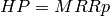The table in Fig. 9.1.3 shows a useful range of power factors for various materials.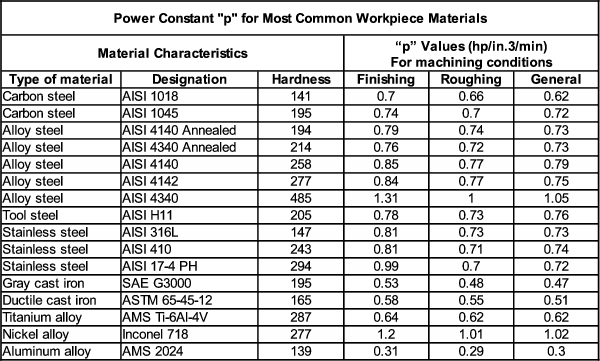Fig. 9.1.3: Metal Removal Power Factors

So here’s a typical turning job. Take a .005” depth of cut on a half-inch diameter 12L14 rod feeding manually at ten inches per minute. How much power does that take?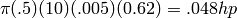How does all this help? Well, clearly if you want to work at increased values of diameter, cutting depth or feed rate, it gives you a way of calculating just how much power that’s likely to take. Note that rotational speed does not enter into the equations at all. So a given job takes the same horsepower whether the work turns at 50, 500 or 5000rpm. This is why engineers refer to lathes, mills and drills as constant horsepower applications. But rotational speed is very important in determining whether or not “enough” horsepower can actually be applied without ruinous chatter.